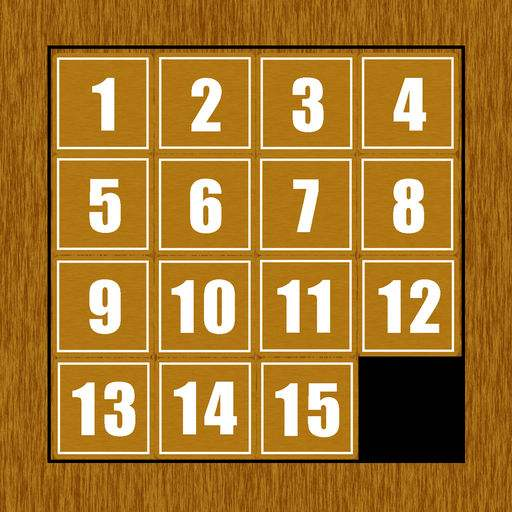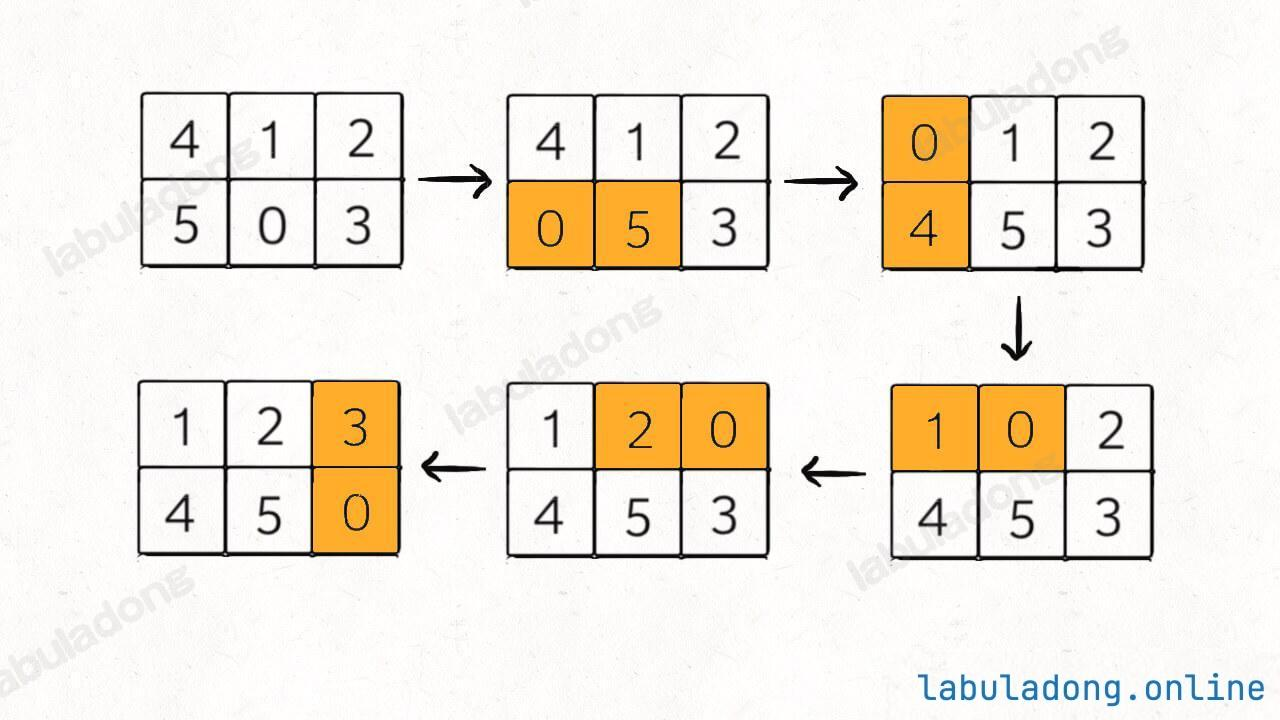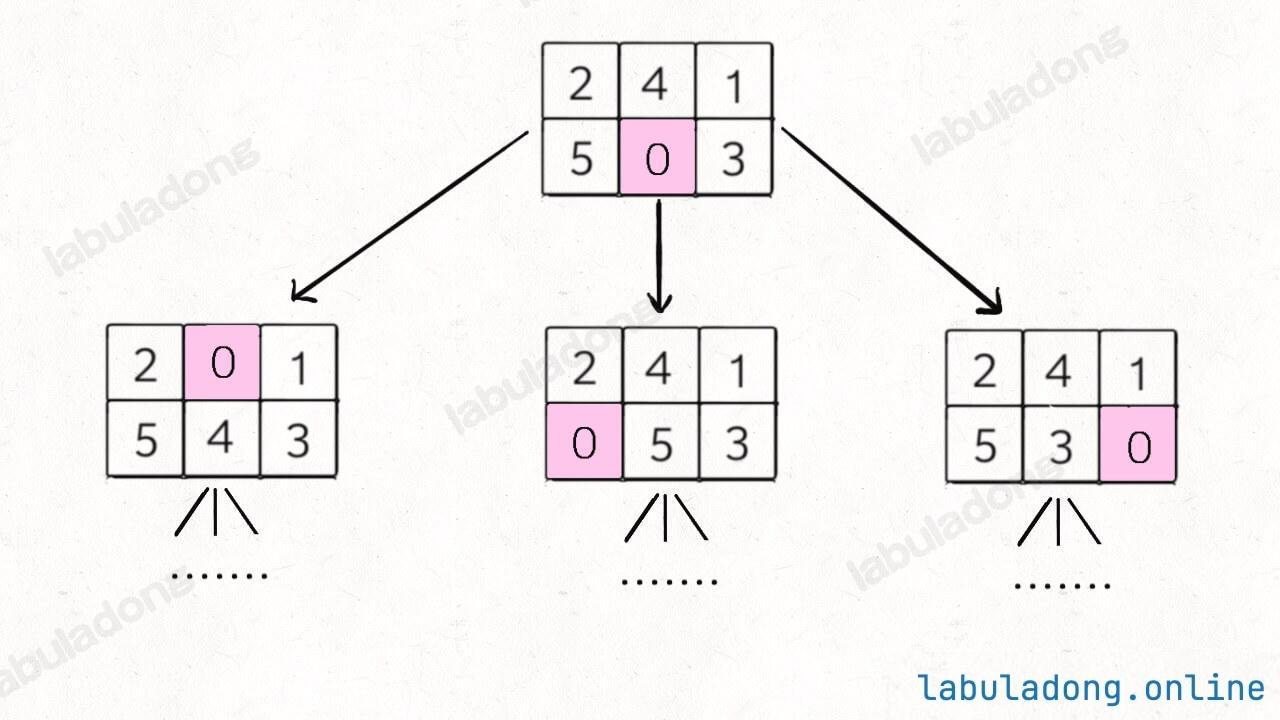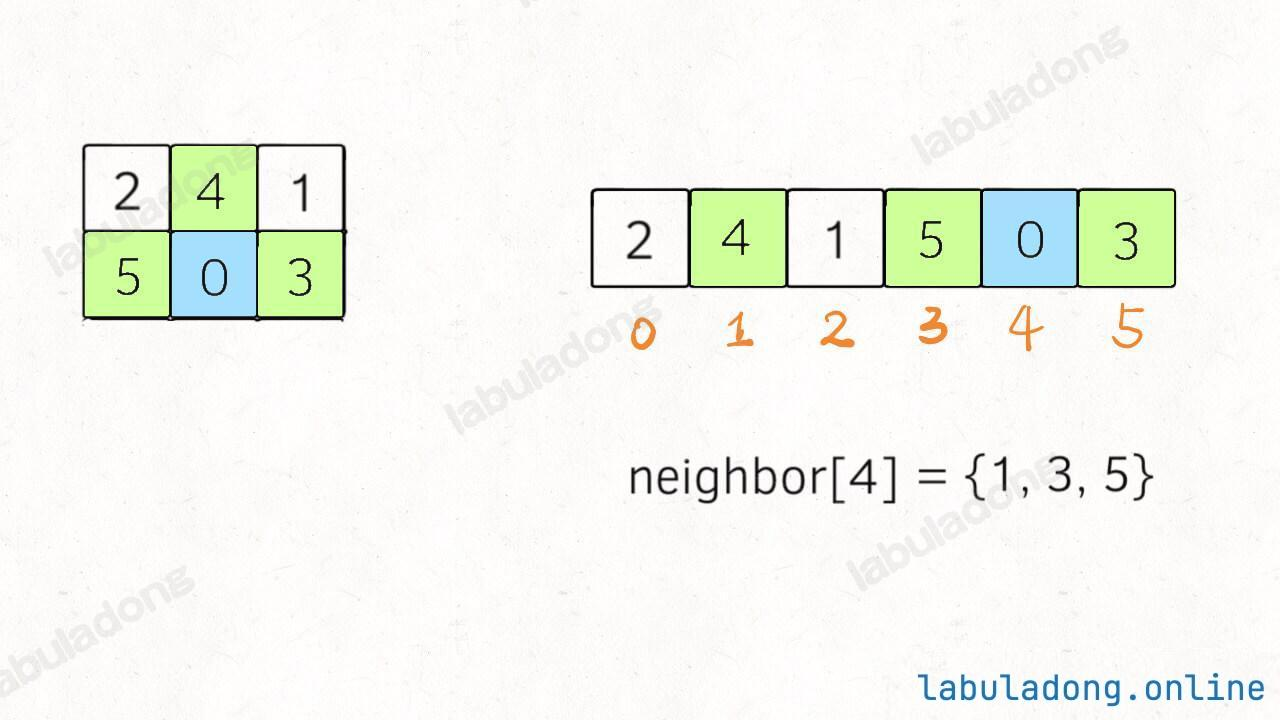Info### # 一、题目解析### # 二、思路分析

1、一般的 BFS 算法，是从一个起点 `start` 开始，向终点 `target` 进行寻路，但是拼图问题不是在寻路，而是在不断交换数字，这应该怎么转化成 BFS 算法问题呢？

2、即便这个问题能够转化成 BFS 问题，如何处理起点 `start` 和终点 `target`？它们都是数组哎，把数组放进队列，套 BFS 框架，想想就比较麻烦且低效。``````// 记录一维字符串的相邻索引
int[][] neighbor = new int[][]{
{1, 3},
{0, 4, 2},
{1, 5},
{0, 4},
{3, 1, 5},
{4, 2}
};
````````````int[][] generateNeighborMapping(int m, int n) {
int[][] neighbor = new int[m * n][];
for (int i = 0; i < m * n; i++) {
List<Integer> neighbors = new ArrayList<>();

// 如果不是第一列，有左侧邻居
if (i % n != 0) neighbors.add(i - 1);
// 如果不是最后一列，有右侧邻居
if (i % n != n - 1) neighbors.add(i + 1);
// 如果不是第一行，有上方邻居
if (i - n >= 0) neighbors.add(i - n);
// 如果不是最后一行，有下方邻居
if (i + n < m * n) neighbors.add(i + n);

// Java 语言特性，将 List 类型转为 int[] 数组
neighbor[i] = neighbors.stream().mapToInt(Integer::intValue).toArray();
}
return neighbor;
}
``````

``````class Solution {
public int slidingPuzzle(int[][] board) {
int m = 2, n = 3;
StringBuilder sb = new StringBuilder();
String target = "123450";
// 将 2x3 的数组转化成字符串作为 BFS 的起点
for (int i = 0; i < m; i++) {
for (int j = 0; j < n; j++) {
sb.append(board[i][j]);
}
}
String start = sb.toString();

// 记录一维字符串的相邻索引
int[][] neighbor = new int[][]{/**<extend up -200><div class="img-content"><img src="/algo/images/sliding_puzzle/4.jpeg" class="myimage"/></div> */
{1, 3},
{0, 4, 2},
{1, 5},
{0, 4},
{3, 1, 5},
{4, 2}
};

/******* BFS 算法框架开始 *******/
HashSet<String> visited = new HashSet<>();
// 从起点开始 BFS 搜索
q.offer(start);

int step = 0;
while (!q.isEmpty()) {/**<extend up -200><div class="img-content"><img src="/algo/images/sliding_puzzle/3.jpeg" class="myimage"/></div> */
int sz = q.size();
for (int i = 0; i < sz; i++) {
String cur = q.poll();
// 判断是否达到目标局面
if (target.equals(cur)) {
return step;
}
// 找到数字 0 的索引
int idx = 0;
for (; cur.charAt(idx) != '0'; idx++) ;
// 将数字 0 和相邻的数字交换位置
for (int adj : neighbor[idx]) {
String new_board = swap(cur.toCharArray(), adj, idx);
// 防止走回头路
if (!visited.contains(new_board)) {
q.offer(new_board);
}
}
}
step++;
}
/******* BFS 算法框架结束 *******/
return -1;
}

private String swap(char[] chars, int i, int j) {
char temp = chars[i];
chars[i] = chars[j];
chars[j] = temp;
return new String(chars);
}
}
``````